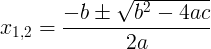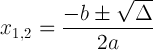# 二次方程

2 + BX + C ^ = 0

x - x 1）（x - x 2）= 0

### 二次公式Δ= b 2 - 4 AC• 当Δ/ 0时，存在2个实根x 1 =（-b + √Δ）/（2a）和x 2 =（-b- √Δ）/（2a）
• 当Δ= 0时，存在一个根x 1 = x 2 = -b /（2a）
• 当Δ<0时，没有实根，则有2个复数根：
x 1 =（-b +i√- Δ）/（2a）和x 2 =（-bi√- Δ）/（2a）

### 问题1

3 x 2 +5 x +2 = 0

#### 解：

a = 3，b = 5，c = 2

X 1,2 =（-5±√（5 2 - 4×3×2））/（2×3）=（-5±√（25-24））/ 6 =（-5±1）/ 6

x 1 =（-5 + 1）/ 6 = -4/6 = -2/3

x 2 =（-5-1）/ 6 = -6/6 = -1

### 问题二

3 x 2 -6 x +3 = 0

#### 解：

a = 3，b = -6，c = 3

X 1,2 =（6±√（（-6）2 - 4×3×3））/（2×3）=（6±√（36-36））/ 6 =（6±0）/ 6

x 1 = x 2 = 1

### 问题三

x 2 +2 x +5 = 0

#### 解：

a = 1，b = 2，c = 5

X 1,2 =（-2±√（2 2 - 4×1×5））/（2×1）=（-2±√（4-20））/ 2 =（-2±√（-16 ））/ 2

x 1 = -1 + 2

x 2 = -1-2 i

### 二次函数图

fx）= ax 2 + bx + c

fx）= 0